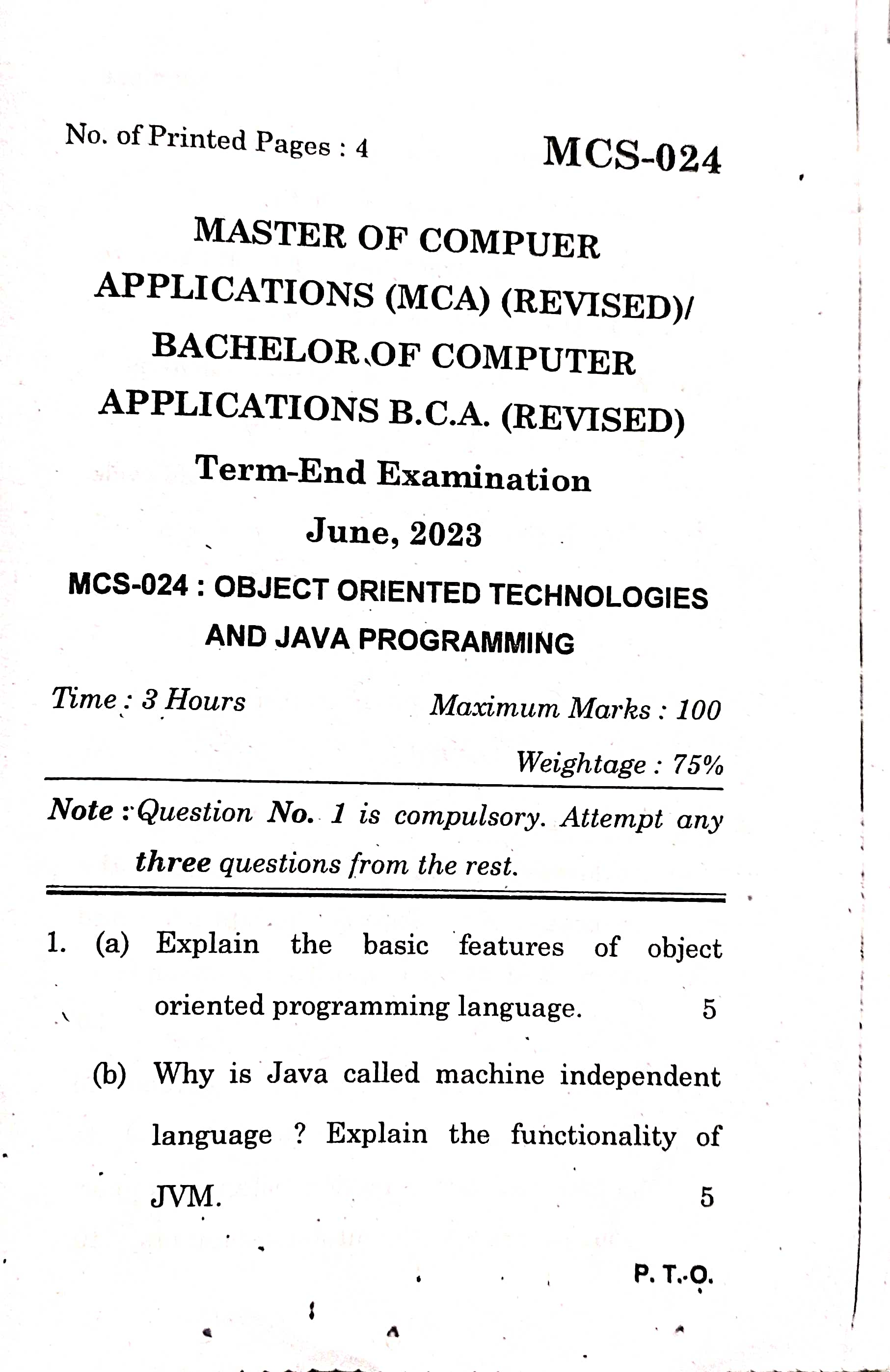# MCS024 : Question 1 (d) Abstract class in Java | June 2023 Paper SolutionQuestion 1. (d) What is an abstract class? Explain the use of abstract class with an example.

An abstract class is like a blueprint for other classes to follow. It cannot be directly used to create objects, but it provides a structure and some common methods that other classes can inherit from. Here's a simple explanation of an abstract class with an example:

The abstract class "Shape" would have properties like "color" and methods like "calculateArea()" and "calculatePerimeter()" that need to be implemented by the actual shape classes. The abstract methods don't have any code in them; they simply declare what needs to be done without specifying how it should be done.

Here's a simplified example:

public abstract class Shape {
protected String color;

public Shape(String color) {
this.color = color;
}

public abstract double calculateArea();
public abstract double calculatePerimeter();

public void displayColor() {
System.out.println("The color of the shape is: " + color);
}
}

In this example, the "Shape" class is an abstract class. It has a constructor to set the color and some methods. The methods "calculateArea()" and "calculatePerimeter()" are declared as abstract, which means that any class inheriting from "Shape" must provide their own implementation of these methods.

Let's say we want to create a "Circle" class that extends the "Shape" class and provides specific calculations for circles. We would implement the abstract methods in the "Circle" class, like this:

public class Circle extends Shape {

public Circle(String color, double radius) {
super(color);
}

@Override
public double calculateArea() {
}

@Override
public double calculatePerimeter() {
return 2 * Math.PI * radius;
}
}

In this "Circle" class, we override the abstract methods to calculate the area and perimeter specifically for circles using the given radius.

By using the abstract class "Shape", we can define a common structure and behavior for shapes while allowing each shape class (like "Circle", "Square", etc.) to implement their own version of the abstract methods. This promotes code reusability, enforces consistency, and provides a convenient way to work with different shapes through a common interface.